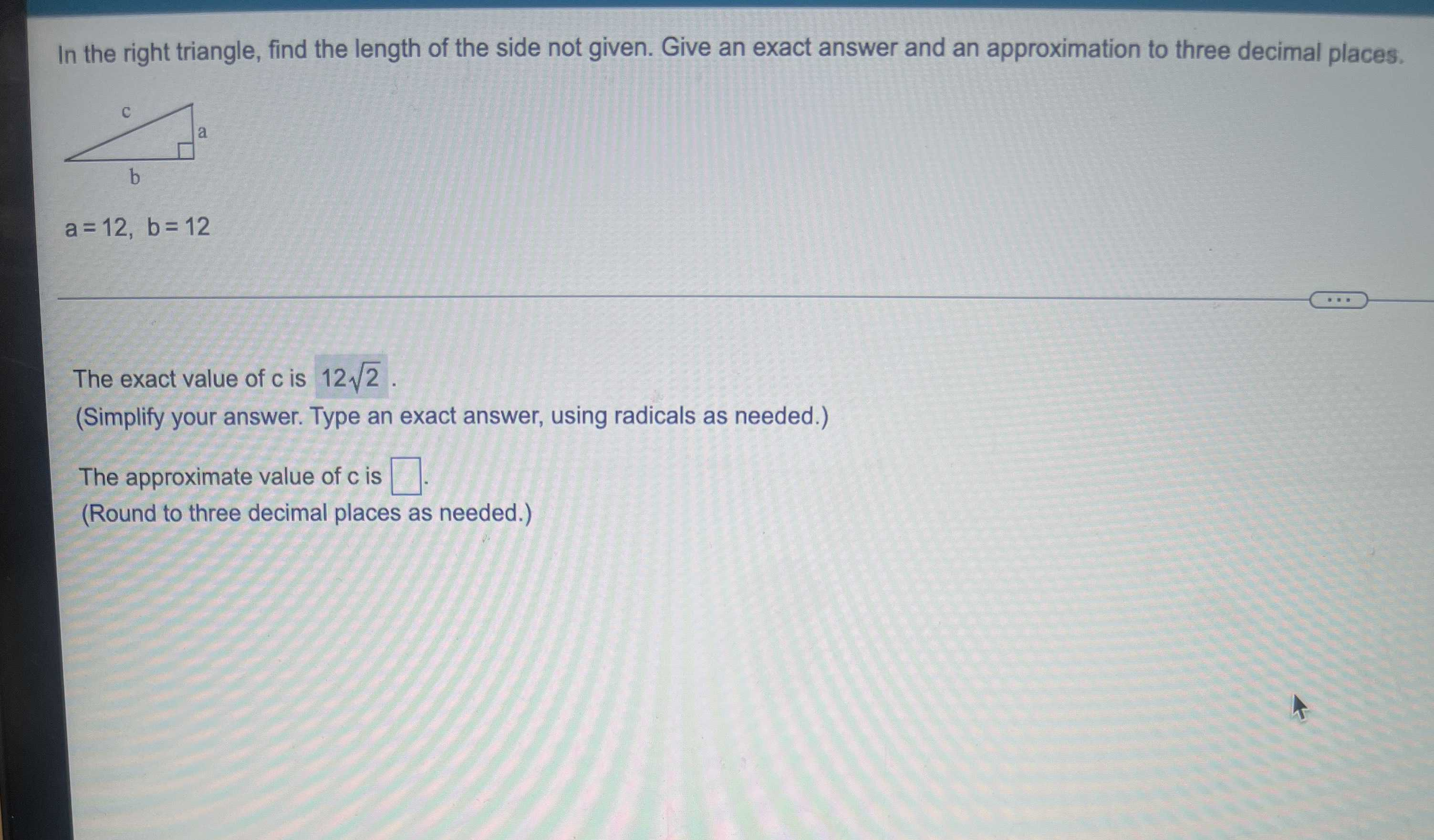### Still have math questions?

Trigonometry
QuestionGive exact answer and an approximation to three decimal places.

In the right triangle, find the length of the side not given. Give an exact answer and an approximation to three decimal places. $$a = 12 , b = 12$$The exact value of $$c$$ is